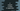# C program to convert a string to uppercase or capitalize all characters## C program to convert a string to uppercase or capitalize all characters:

This program will show you how to convvert a string to uppercase. For storing a string, we use one character array. Our program will ask the user to enter a string. It will store that string in the array and then convert all character to uppercase. We can solve this program in two ways. We can write our own code to do the conversion or we can use a library function that is already available for this. I will show you both of these ways.

### Method 1: C program to convert a string to uppercase using a function:

The ASCII values of A, a, z and Z are:

``````a - 97
z - 122
A - 65
Z - 90``````

We will check if a character is equal to or greater than a and less than or equal to z. If yes, we will decrement its value by 97 - 65 = 32.

Below is the complete C program that uses the above logic:

``````#include <stdio.h>
#include <string.h>

void convertToUppercase(char *givenStr)
{
int i;
for (i = 0; givenStr[i] != '\0'; i++)
{
if (givenStr[i] >= 'a' && givenStr[i] <= 'z')
{
givenStr[i] = givenStr[i] - 32;
}
}
}

int main()
{
char givenStr;

printf("Enter a string : \n");
fgets(givenStr, 100, stdin);

convertToUppercase(givenStr);
puts(givenStr);

return 0;
}``````

### Explanation:

Here,

• givenStr is a character array to store the user given string. We are reading that string and storing that in the variable givenStr.
• convertToUppercase method is used to convert one given string to uppercase. We can pass one string to this method and it iterates through the characters one by one and move each to upper case.
• convertToUppercase uses the same logic that we discussed before i.e. it decrements each character by 32.
• Once the upper case conversion is done, we are printing the string on console using puts.

### Sample output:

Let’s take a look at the below outputs for the above program:

``````Enter a string :
the quick brown fox jumps over the lazy dog
THE QUICK BROWN FOX JUMPS OVER THE LAZY DOG

Enter a string :
The Quick Brown Fox Jumps Over The Lazy Dog
THE QUICK BROWN FOX JUMPS OVER THE LAZY DOG``````

As you can see here, it capitalize all characters.

### Method 2: Using toUpper:

toUpper is another method used to convert one lowecase letter to uppercase. We can use this method to all characters of the string. Still, we need the loop. Below program uses toUpper:

``````#include <stdio.h>
#include <string.h>

void changeString(char *givenStr)
{
int i;
for (i = 0; givenStr[i] != '\0'; i++)
{
givenStr[i] = toupper(givenStr[i]);
}
}

int main()
{
char givenStr;

printf("Enter a string : \n");
fgets(givenStr, 100, stdin);

changeString(givenStr);
puts(givenStr);

return 0;
}``````

It prints similar output as the above one.

``````Enter a string :
hello world
HELLO WORLD``````